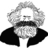## Where communities thrive

• Join over 1.5M+ people
• Join over 100K+ communities
• Free without limits
##### Repo info
•••••••••••••••••••••##### Activityeykiriku
@eykiriku
in short, yes you were rightJaakko Luttinen
@jluttine
if you observe probabilities, maybe one can use beta or dirichlet node. if you have noisy observations of a categorical variable, maybe you can add a noisy observed categorical variable that has the true (unknown) state as a parent.
s/probabilities/probabilities or some other variables that are positive and sum to one/eykiriku
@eykiriku
true, thankseykiriku
@eykiriku
my problem was more related to bayespy, like assume i choose a dirichlet observation node Y. Y has 2 states, Normal and High. As above, any evidence will belong to the array [0, 5] and the fuzzy rules may lead to an output saying 80% activation of high and 20%.... My doubt is how to define the variable 'activity', the one to be observed for inference in Y.observe(activity), using this fuzzy ouput@jluttine , Hi! Maybe you know why I can't fix the seed of the random number generator with np.random.seed()? Is it not the seed that must fix? I'm trying to obtain the same results in likelihood in different runs without success... :Sit seems silly, but I can't find a way in django tests to produce the same inference results, because neither np.random.seed or random.seed seems to work... :Sseems that PYTHONHASHSEED=number makes it workJaakko Luttinen
@jluttineMarvie Demit
hi @jluttine I am starting to use bayespy and I want to make a wrapper function fitting gaussian mixture for model selection.
def fitted_gaussian(N, n_krnl, D, covariance = 'full'):
# Input:
# N = Number of data vectors
# D = Dimensionality
# n_krnl = Number of kernels
# Prior
P = nds.Dirichlet(1e-5*np.ones(n_krnl), name='P')
# N n_krnl-dimensional cluster (for the data)
I = nds.Categorical(P, plates=(N,), name='I')
# n_krnl D-dimensional components means
if covariance == 'full':
# n_krnl D-dim component covariance
mu = nds.Gaussian(np.zeros(D), 1e-5*np.identity(D), plates = (n_krnl,), name = 'mu')
Lambda = nds.Wishart(D, 1e-5*np.identity(D), plates = (n_krnl,), name = 'Lambda')
Y = nds.Mixture(I, nds.Gaussian, mu, Lambda, plates = (N,),  name = 'Y')
else:
print('diagonal')
# inverse variances
mu = nds.GaussianARD(np.zeros(D), 1e-5*np.identity(D), shape = (D,), plates = (n_krnl,), name = 'mu')
Lambda = nds.Gamma(1e-3, 1e-3, plates = (n_krnl, D), name = 'Lambda')
Y = nds.Mixture(I, nds.GaussianARD, mu, Lambda, plates = (N,),  name = 'Y')
I.initialize_from_random()
return VB(Y, mu, Lambda, I, P)
the problem is that I get this when I apply it with the diagonal; ValueError: The plates (2,) of the parents are not broadcastable to the given plates (10,).
How can I fix this?Jaakko Luttinen
@jluttineJaakko Luttinen
@jluttine
Don_Chili_twitter: can't really test now but i guess the issue is that GaussianARD makes scalar gaussian variables by default. in principle, you should be able to fix it by giving ndim=1 to Mixture but i think it doesn't work atm. alternatively, you can create a diagonal matrix from Lambda by Lambda.as_diagonal_wishart() and using nds.Gaussian instead of nds.GaussianARD in
Mixture
that is: Y = nds.Mixture(I, nds.Gaussian, mu, Lambda.as_diagonal_wishart(), plates=(N,), name='Y')
couldn't test thoughMarvie Demit
Hi @jluttine ! Thank you for your very fast response: n_krnl should be 10. I already tried your recommendation but there is something weird about the plates, although I already tried to make the parent plates the same dimension as the given plates but it never match......ValueError: The plates (2,) of the parents are not broadcastable to the given plates (10,).Jaakko Luttinen
@jluttine
ok, i'll need to check that with actual python. maybe tomorrow@jluttine are you there?Jaakko Luttinen
@jluttine
yep:)
Hi Jaako, can I ask you for a quick review on a text?Jaakko Luttinen
@jluttineI'm finishing the documentation of the first release of django-ai and I wanted your opinion - if I am missing somethingJaakko Luttinen
@jluttine
okJaakko Luttinen
@jluttine
student t distribution is possible with bayespy. one just needs to construct it as a combination of gamma+gaussian. so one could implement mixture of student t with bayespyah, cool, how would that be?Jaakko Luttinen
@jluttine
section 2Jaakko Luttinen
@jluttine
yep
vb learning can converge to bad local minima. better initialization or better learning algorithm may help. for instance, deterministic annealing. also, sometimes changing the model might help. for instance, if one factors q(mu)q(Lambda), then re-formulating so that one gets q(mu,Lambda) might improve the posterior accuracy and the learningaha, then I will change it to something like "implementing a student t distribution would require extra complexity which is out of the scope of this example"Jaakko Luttinen
@jluttine
yepdoesn't the mixture node needs a class as a parameter not a network?
for the case of the constructing the t for the mixtureJaakko Luttinen
@jluttine
not sure what you mean, but the mixture in a mixture model is a different mixture than in student t construction. student t construction is based on an infinite mixture. it's basically just a particular gaussian-gamma joint distribution with the gamma distribution marginalized. but in vb approach, one doesn't marginalize the gamma analytically in order to keep the equations in
the exponential family form
i should write an example
some daywhat I thought was using two nodes to represent the t, but that won't do as an input to the mixture node... I just glanced at the links and the topic, it is new to me :)
I'm in a hurry for the release, so i'll postpone it
an example would be great :)
thanks a lot, Jaakko!Jaakko Luttinen
@jluttine
npDingBoom
@DingBoom
Hi @jluttine !I want to know is this result can only be shown by graph?Can we show probability density just like a format or some text?Thanks a lot!Ludvins
@Ludvins
Hello, I'm trying to learn a Gaussian mixture from Mnist but I get "ValueError: Must pass 2-d input", can't I use higher dimensional data?